# 深度学习笔记（二十二）EfficientDet

TensorFlow 实现

PyTorch 实现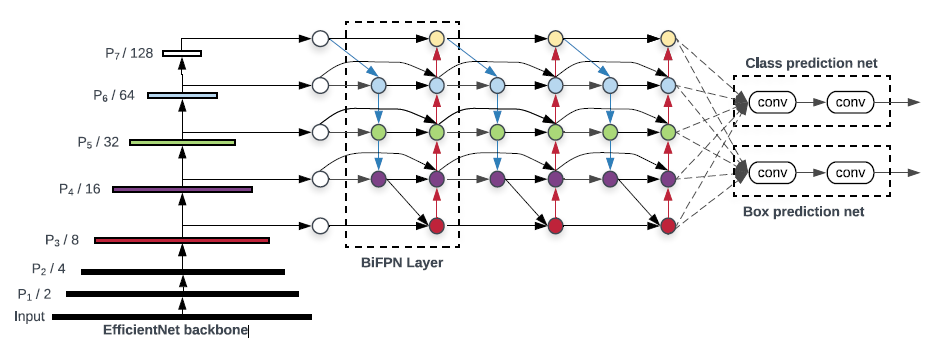EfficientDet 是目前最优秀的检测器，backbone 是基于 depthwise separable convolution 和 SE 模块利用 AutoML 搜索出来的，EfficientDet 出彩的地方在于设计了高效的 FPN 结构，即 BiFPN。

# 摘要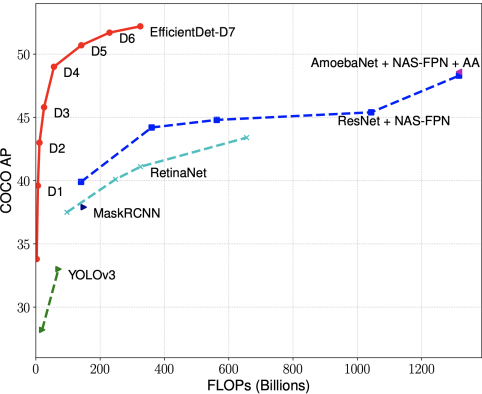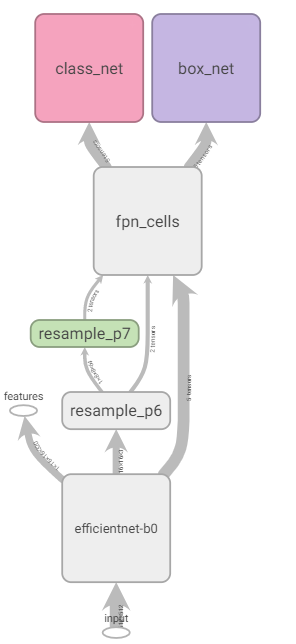backbone+fpn+box params/flops = 3.880067M, 2.535978423B

# EfficientNet

# Coefficients:   width,depth,res,dropout
'efficientnet-b0': (1.0, 1.0, 224, 0.2),
'efficientnet-b1': (1.0, 1.1, 240, 0.2),
'efficientnet-b2': (1.1, 1.2, 260, 0.3),
'efficientnet-b3': (1.2, 1.4, 300, 0.3),
'efficientnet-b4': (1.4, 1.8, 380, 0.4),
'efficientnet-b5': (1.6, 2.2, 456, 0.4),
'efficientnet-b6': (1.8, 2.6, 528, 0.5),
'efficientnet-b7': (2.0, 3.1, 600, 0.5)

1. 由 width 参数控制除 MBConvBlock 外两个卷积层（Stage1 和 Stage9）的输出通道数:def round_filters(filters, global_params):
""" Calculate and round number of filters based on depth multiplier. """
multiplier = global_params.width_coefficient
if not multiplier:
return filters
divisor = global_params.depth_divisor # default = 8
min_depth = global_params.min_depth
filters *= multiplier
min_depth = min_depth or divisor       # min_depth default is None
new_filters = max(min_depth, int(filters + divisor / 2) // divisor * divisor)
if new_filters < 0.9 * filters:  # prevent rounding by more than 10%
new_filters += divisor
return int(new_filters)
View Code

2. 由 depth 控制 Block 重复次数(向上取整):def round_repeats(repeats, global_params):
""" Round number of filters based on depth multiplier. """
multiplier = global_params.depth_coefficient
if not multiplier:
return repeats
return int(math.ceil(multiplier * repeats))
View Code

3. res 控制图像输入尺度，dropout 控制最后 AVG_POOL 和 FC 之间的 DropOut 层的 dropout_rate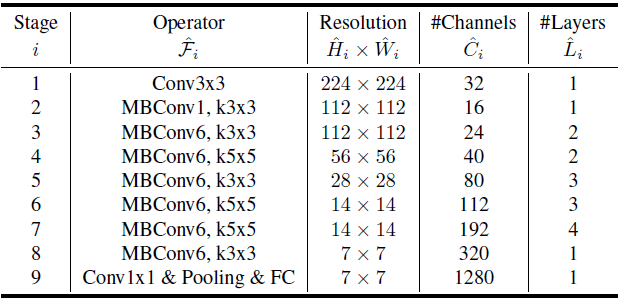# Stem Stage1 x = self._swish(self._bn0(self._conv_stem(inputs))) # Blocks Stage2-8 for idx, block in enumerate(self._blocks): drop_connect_rate = self._global_params.drop_connect_rate if drop_connect_rate: drop_connect_rate *= float(idx) / len(self._blocks) x = block(x, drop_connect_rate=drop_connect_rate) # Head Stage9_1 x = self._swish(self._bn1(self._conv_head(x))) # Pooling and final linear layer Stage9_2 x = self._avg_pooling(x) x = x.view(bs, -1) x = self._dropout(x) x = self._fc(x)

# Expansion and Depthwise Convolution
x = inputs
if self._block_args.expand_ratio != 1:
x = self._expand_conv(inputs)
x = self._bn0(x)
x = self._swish(x)

x = self._depthwise_conv(x)
x = self._bn1(x)
x = self._swish(x)

# Squeeze and Excitation
if self.has_se:
x_squeezed = self._se_reduce(x_squeezed)
x_squeezed = self._swish(x_squeezed)
x_squeezed = self._se_expand(x_squeezed)
x = torch.sigmoid(x_squeezed) * x

x = self._project_conv(x)
x = self._bn2(x)

# Skip connection and drop connect
input_filters, output_filters = self._block_args.input_filters, self._block_args.output_filters
if self.id_skip and self._block_args.stride == 1 and input_filters == output_filters:
if drop_connect_rate:
x = drop_connect(x, p=drop_connect_rate, training=self.training)
x = x + inputs  # skip connection

# BiFPN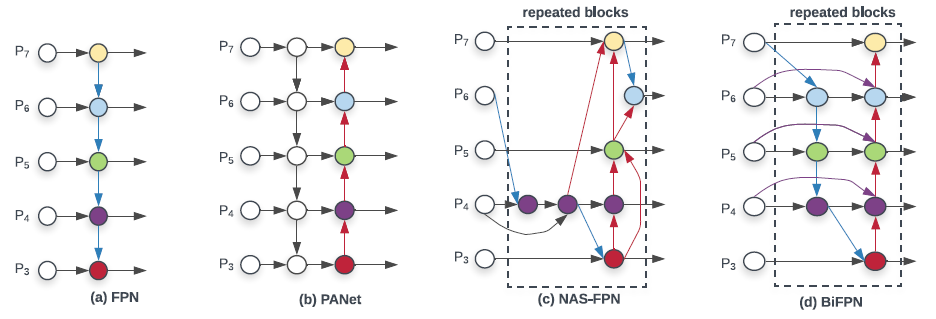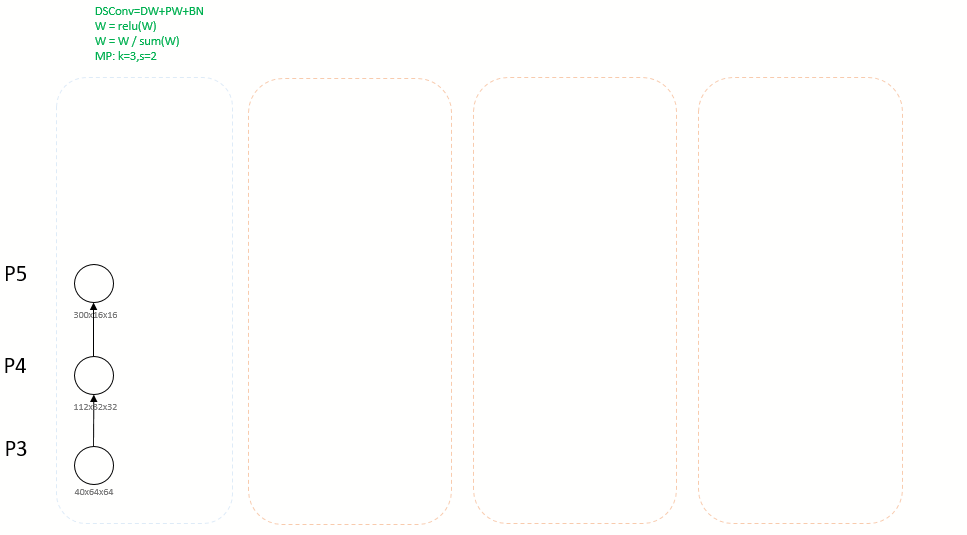BiFPN 相比 FPN 能涨 4 个点，而且参数量反而是下降的：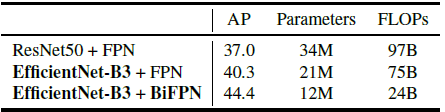# Anchor

\begin{equation}
\label{Anchor}
\begin{split}
& anchor\_scale = 4.0 \\
& strides = [8, 16, 32, 64, 128] \\
& scales = [2^0, 2^{\frac{1}{3}}, 2^{\frac{2}{3}}] \\
& ratios = [(1.0, 1.0), (1.4, 0.7), (0.7, 1.4)] \\
& base\_anchor\_size = anchor\_scale * stride * scale  \\
& anchor\_size\_w = base\_anchor_size * ratio  \\
& anchor\_size\_h = base\_anchor_size * ratio
\end{split}
\end{equation}

# Regressor & Classifier

## Anchor+Regression

YOLOV3 编解码倒是很像，区别在于中心点偏移没有经过 sigmoid 函数：

\begin{equation}
\label{a}
\begin{split}
& b_x =  t_x + c_x \\
& b_y =  t_y + c_y \\
& b_w =  p_w e^{t_w} \\
& b_h =  p_h e^{t_h} \\
\end{split}
\end{equation}

# LOSS

## 回归目标

\begin{equation}
\label{target}
\begin{split}
& \delta_x = (g_x - c_x) / p_w \\
& \delta_y = (g_y - c_y) / p_h \\
& \delta_w = log(g_w / p_w) \\
& \delta_h = log(g_h / p_h) \\
\end{split}
\end{equation}

\begin{equation}
\label{Smooth_L1}
smooth_{L_1}(x) = \begin{cases}
\ 0.5 * 9.0 * x^2 & if \ \lvert{x}\rvert < 1.0/9.0 \\
\ \lvert{x}\rvert - 0.5/9.0 & \ otherwise
\end{cases}
\end{equation}

# Compound Scaling

Model Scaling 指的是人们经常根据资源的限制，对模型进行调整。比如说为了把 backbone 部分 scale up，得到更大的模型，就会考虑把层数加深， Res50 -> Res101这种，或者说比如把输入图的分辨率拉大。

EfficientNet 在 Model Scaling 的时候考虑了网络的 width, depth, and resolution 三要素。而 EfficientDet 进一步扩展, 把 EfficientNet 拿来做 backbone 的同时，neck 部分，BiFPN 的 channel 数量重复的 layer 数量也可以控制；此外还有 head 部分的层数，以及 输入图片的分辨率，这些组成了 EfficientDet 的 scaling config 。

self.backbone_compound_coef = [0, 1, 2, 3, 4, 5, 6, 6]
self.fpn_num_filters = [64, 88, 112, 160, 224, 288, 384, 384]
self.fpn_cell_repeats = [3, 4, 5, 6, 7, 7, 8, 8]
self.input_sizes = [512, 640, 768, 896, 1024, 1280, 1280, 1536]
self.box_class_repeats = [3, 3, 3, 4, 4, 4, 5, 5]

# Q

### 9. 数据增广太单一，只有随机翻转

posted @ 2020-04-22 10:10  xuanyuyt  阅读(369)  评论(0编辑  收藏